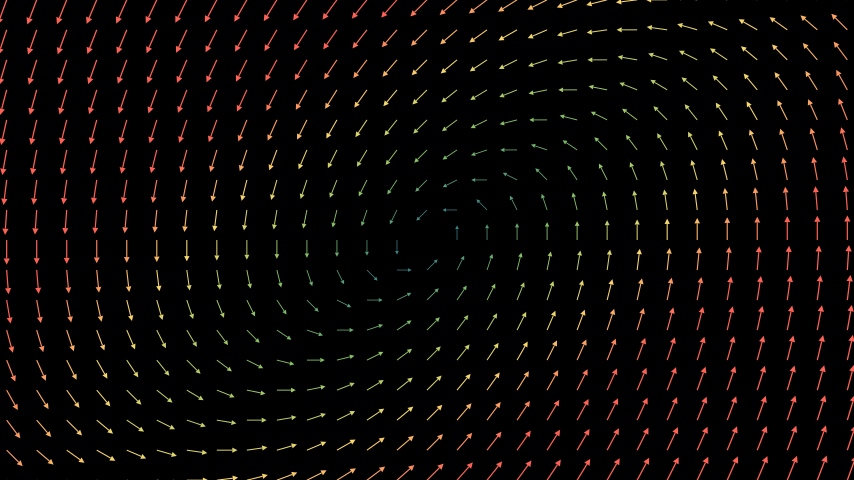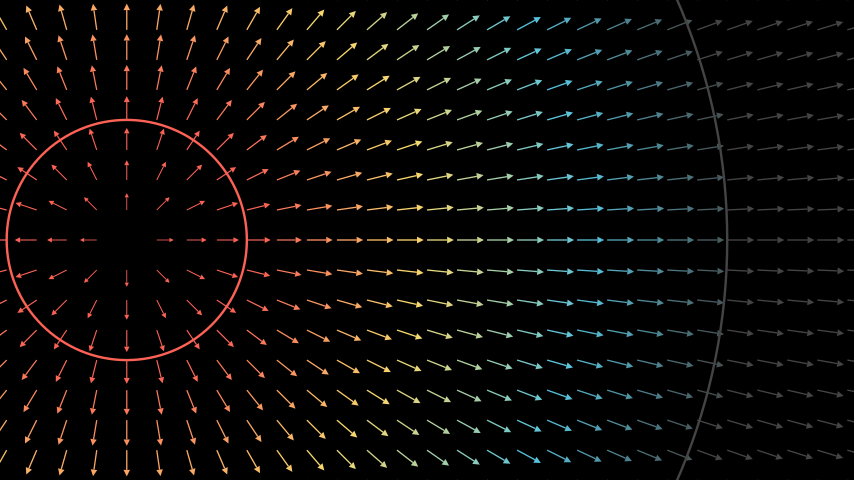# ArrowVectorField#

Qualified name: `manim.mobject.vector\_field.ArrowVectorField`

class ArrowVectorField(func, color=None, color_scheme=None, min_color_scheme_value=0, max_color_scheme_value=2, colors=['#236B8E', '#83C167', '#FFFF00', '#FC6255'], x_range=None, y_range=None, z_range=None, three_dimensions=False, length_func=<function ArrowVectorField.<lambda>>, opacity=1.0, vector_config=None, **kwargs)[source]#

Bases: `VectorField`

A `VectorField` represented by a set of change vectors.

Vector fields are always based on a function defining the `Vector` at every position. The values of this functions is displayed as a grid of vectors. By default the color of each vector is determined by it’s magnitude. Other color schemes can be used however.

Parameters
• func (Callable[[np.ndarray], np.ndarray]) – The function defining the rate of change at every position of the vector field.

• color (Color | None) – The color of the vector field. If set, position-specific coloring is disabled.

• color_scheme (Callable[[np.ndarray], float] | None) – A function mapping a vector to a single value. This value gives the position in the color gradient defined using min_color_scheme_value, max_color_scheme_value and colors.

• min_color_scheme_value (float) – The value of the color_scheme function to be mapped to the first color in colors. Lower values also result in the first color of the gradient.

• max_color_scheme_value (float) – The value of the color_scheme function to be mapped to the last color in colors. Higher values also result in the last color of the gradient.

• colors (Sequence[Color]) – The colors defining the color gradient of the vector field.

• x_range (Sequence[float]) – A sequence of x_min, x_max, delta_x

• y_range (Sequence[float]) – A sequence of y_min, y_max, delta_y

• z_range (Sequence[float]) – A sequence of z_min, z_max, delta_z

• three_dimensions (bool) – Enables three_dimensions. Default set to False, automatically turns True if z_range is not None.

• length_func (Callable[[float], float]) – The function determining the displayed size of the vectors. The actual size of the vector is passed, the returned value will be used as display size for the vector. By default this is used to cap the displayed size of vectors to reduce the clutter.

• opacity (float) – The opacity of the arrows.

• vector_config (dict | None) – Additional arguments to be passed to the `Vector` constructor

• kwargs – Additional arguments to be passed to the `VGroup` constructor

Examples

Example: BasicUsage```from manim import *

class BasicUsage(Scene):
def construct(self):
func = lambda pos: ((pos * UR + pos * LEFT) - pos) / 3
```

Example: SizingAndSpacing

```from manim import *

class SizingAndSpacing(Scene):
def construct(self):
func = lambda pos: np.sin(pos / 2) * UR + np.cos(pos / 2) * LEFT
vf = ArrowVectorField(func, x_range=[-7, 7, 1])
self.wait()

length_func = lambda x: x / 3
vf2 = ArrowVectorField(func, x_range=[-7, 7, 1], length_func=length_func)
self.play(vf.animate.become(vf2))
self.wait()
```

Example: Coloring```from manim import *

class Coloring(Scene):
def construct(self):
func = lambda pos: pos - LEFT * 5
colors = [RED, YELLOW, BLUE, DARK_GRAY]
vf = ArrowVectorField(
func, min_color_scheme_value=2, max_color_scheme_value=10, colors=colors
)
```

Methods

 `get_vector` Creates a vector in the vector field.

Attributes

 `animate` Used to animate the application of any method of `self`. `animation_overrides` `color` `depth` The depth of the mobject. `fill_color` If there are multiple colors (for gradient) this returns the first one `height` The height of the mobject. `n_points_per_curve` `sheen_factor` `stroke_color` `width` The width of the mobject.
get_vector(point)[source]#

Creates a vector in the vector field.

The created vector is based on the function of the vector field and is rooted in the given point. Color and length fit the specifications of this vector field.

Parameters

point (ndarray) – The root point of the vector.